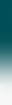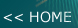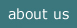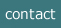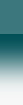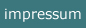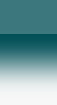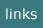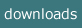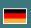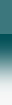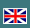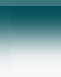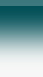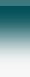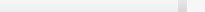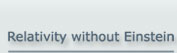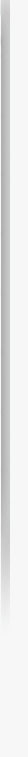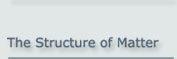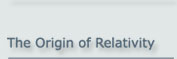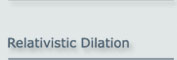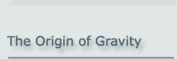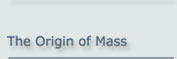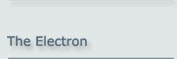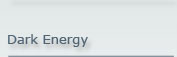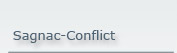The Structure of Matter: The Basic Particle Model

A fundamental particle model explains Newton physics,
relativity (SR and GR), duality, and particle properties in a classical way.

Summary

A particle model, which is assumed to be applicable for elementary particles, i.e. for

- Leptons
- Quarks

explains - besides classical mechanics - phenomena which are normally contributed to relativity (SR and GR) and to quantum mechanics. The model will be called the "Basic Particle Model".

The following physical phenomena are consequences of this particle model

- Inertial mass
- Relativistic increase of the mass
- Mass-energy equivalence
- Newton's law of motion
- Energy conservation
- Constancy of the angular momentum (spin)
- Magnetic moment
- Gyro-magnetic relation
- Relativistic dilation of time
- Gravity
- Relativistic change of gravity
- Particle-wave duality.

And please note: The consequences listed here are not merely philosophical ideas, but are proven by quantitatively correct results!

(Note: This site is also available as a pdf file.)

1 Introduction

According to the Basic Particle Model, every elementary particle is built by 2 mass-less constituents which orbit each other with the speed of light c. The frequency of the circulation is the deBroglie frequency (Figure 1.1).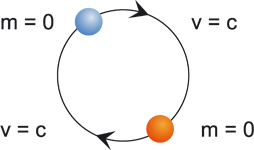Figure 1.1: Structure of an elementary particle according to the Basic Particle Model

2 History of the Basic Particle Model

The Basic Particle Model can be related to the work of de Broglie, Dirac, and Schrödinger in the 1920ies.
1st Louis de Broglie has stated (1923) that every elementary particle has an internal oscillation, the frequency ν of which is related to the gross energy E of the particle by the relation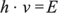(2.1)

where h is the Planck constant .

2nd Paul Dirac has developed the relativistic wave function of the Electron which was analysed by Schrödinger.

3rd Erwin Schrödinger. He found that, as a consequence of the Dirac function, the inside of the electron permanently moves with the speed of light c.

These assumptions in a synopsis caused some physical problems:

• Already at the time of Dirac and Schrödinger it was assumed that the electron is a point-like object which has no further constituents
Consequence:
A point like object cannot oscillate because this would mean a permanent violation of the momentum law

• The electron has a mass
Consequence: No motion with the speed of light c is possible. This would be a violation of special relativity.

These conflicts were not resolved during the past 80 years but they were related to quantum mechanics which by the current common sense cannot be understood by imagination. This position was excepted by most members of the physical community. But, in contrast to this common sense, the matter can in fact be understood by imagination if few assumptions are made which are very natural:

• We have to assume that the electron is built by 2 constituents

• These constituents are mass-less and orbit each other at the speed of light c

These assumptions solve the problems named above:

• There is no momentum conflict any longer

• For a mass-less object the motion at the speed of light is natural.

And on the other hand there are no conflicts with the current experimental situation of physics:

• The conclusion that the electron has no constituents was taken from scattering experiments. The evaluation of these experiment has always supposed that, if the electron should have constituents, these should have a mass on their own. The alternative assumption of 'no mass' was never taken into account. The full rational used here is explained in the context of the ELECTRON.

• The origin of the mass of the electron in its entity is caused by the fact that any configuration of objects bound to each other in a way, that they maintain a distance to each other, has an inertial behaviour. The is explained in the context of the INERTIAL MASS.

The structure of an elementary particle described above is assumed to be valid for every lepton and every quark. It is called the "Basic Particle Model".

3 Consequences of the Basic Particle Model

The Basic Particle Model is a powerful model which is able to make a lot of physical phenomena understandable. These phenomena are listed in the abstract above. (These phenomena are normally explained by different assumptions of "physical principles" and other assumed fundamental laws, which normally have no further explanation and which are partially even in conflict to each other.)

3.1 Consequences with Respect to Relativity

For the case of special relativity the phenomenon of dilation is a consequence of the model. Details are in the given links about special RELATIVITY in general and about TIME DILATION.

3.2 Consequences with Respect to Inertial Mass

The inertial mass of an elementary particle and the mass to size relation is a consequence of the model. From this application of the model there also follows the relativistic increase of mass at motion and the mass-energy equivalence. In addition Newton's law of motion is a consequence of the particle structure assumed in this model, and, as a further consequence of Newton's law, the law of energy conservation. This is explained in the context of the INERTIAL MASS.

3.3 Consequences with Respect to Gravity

Following an idea of Roman Sexl, General Relativity can be explained using the refraction of light-like particles at a gravitational potential. This concept in connection with the basic particle model is able to explain GRAVITY or gravity and the other phenomena related to General Relativity.

3.4 Consequences with Respect to Particle Properties

Particle properties, which by physical common sense can only be described by quantum mechanics, can be classically deduced by the basic particle model. This is explained for the example of the ELECTRON, but can be applied to all elementary particles. This covers the constancy of the spin, the magnetic moment, and also the mass-dependency of the gyro-magnetic relation.

3.5 Particle-Wave Duality

The particle-wave duality, which is the principle pile of quantum mechanics (within the so called 'Copenhagen interpretation') can, following Louis de Broglie, be classically understood in the view of the basic particle model. This is explained (in this case qualitatively) in the context of SPECIAL RELATIVITY (last section).

4 References

` Louis de Broglie, RADIATIONS - Ondes et Quanta, Comptes rendus, Vol. 177, 1923, pp. 507-510.`

NOTE:

The concept of the "Basic Particle Model" of matter was presented initially at the Spring Conference of the German Physical Society (Deutsche Physikalische Gesellschaft) on 24 March 2000 in Dresden

by Albrecht Giese.

2021-05-15